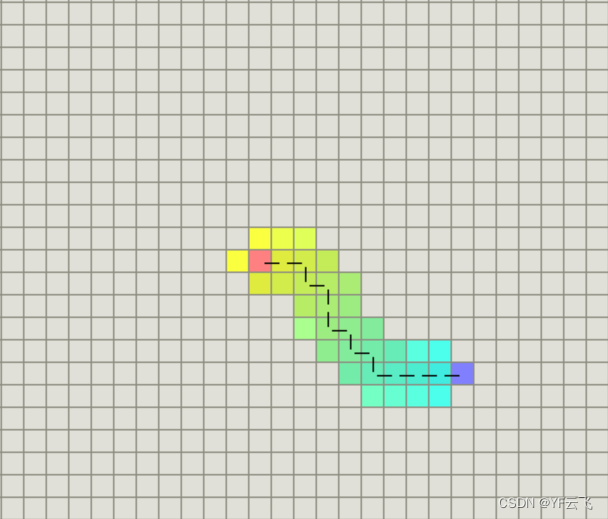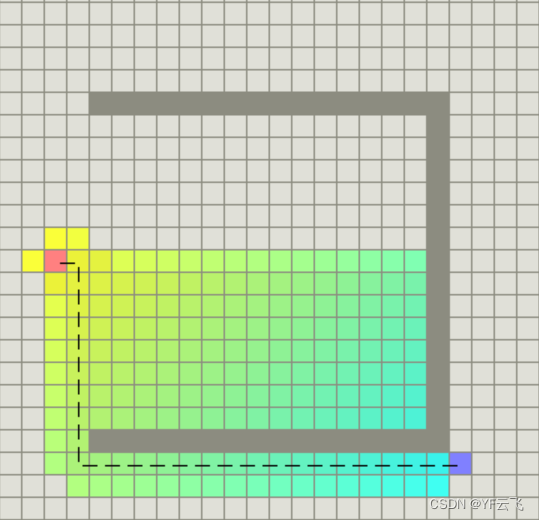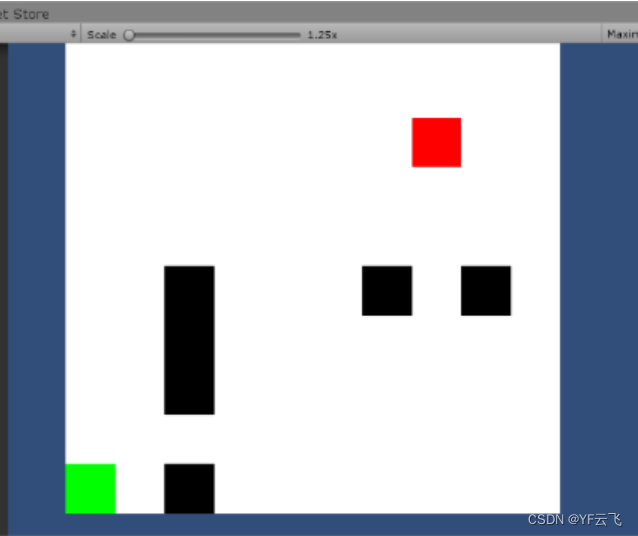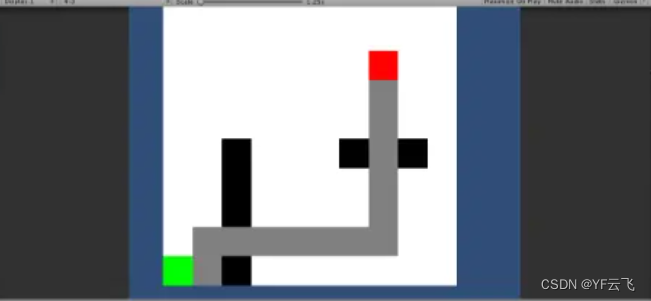## 前言

### A* 算法的基本概念A* 在从起点移动到目标时平衡两者。每次通过主循环，它检查 n具有最低的顶点 `f(n) = g(n) + h(n)`

### A* 算法的实现原理

A算法的基本思想就是借助这些网格实现寻路，从起点开始遍历四周的点，寻找最有可能在最短路径上的点，并以这个点为基准继续向四周遍历，直至遍历到终点，路径也就找到了。

（1）如果这个点是障碍，直接无视。
（2）如果这个点不在 open 表和 close 表中，则加入 open 表
（3）如果这个点已经在 open 表中，并且当前基点所在路径代价更低，则更新它的 G 值和父亲
（4）如果这个点在 close 表中，忽略。

## 正文

### A* 算法实现

``````public class Point
{
public int X;
public int Y;
public int F;
public int G;
public int H;
public Point parent=null;

public bool isObstacle = false;

public Point(int x,int y)
{
X = x;
Y = y;
}

public void SetParent(Point parent,int g)
{
this.parent = parent;
G = g;
F = G + H;
}
}``````

``````using System.Collections;
using System.Collections.Generic;
using UnityEngine;

public class AStar : MonoBehaviour
{
public const int width = 10;
public const int height = 10;

public Point[,] map = new Point[height,width];
public SpriteRenderer[,] sprites = new SpriteRenderer[height, width];//图片和结点一一对应

public GameObject prefab;   //代表结点的图片
public Point start;
public Point end;

void Start()
{
InitMap();
//测试代码
SetStartAndEnd(0, 0, 7, 7);
FindPath();
ShowPath();
}

public void InitMap()//初始化地图
{
for(int i=0;i<width;i++)
{
for (int j = 0; j < height; j++)
{
sprites[i, j] = Instantiate(prefab, new Vector3(i, j, 0),Quaternion.identity).GetComponent<SpriteRenderer>();
map[i, j] = new Point(i, j);
}
}
}

{
map[x, y].isObstacle = true;
sprites[x, y].color = Color.black;
}

public void SetStartAndEnd(int startX,int startY,int endX,int endY)//设置起点和终点
{
start = map[startX,startY];
sprites[startX, startY].color = Color.green;
end = map[endX, endY];
sprites[endX, endY].color = Color.red;
}

public void ShowPath()//显示路径
{
Point temp = end.parent;
while(temp!=start)
{
sprites[temp.X, temp.Y].color = Color.gray;
temp = temp.parent;
}
}

public void FindPath()
{
List<Point> openList = new List<Point>();
List<Point> closeList = new List<Point>();
while(openList.Count>0)//只要开放列表还存在元素就继续
{
Point point = GetMinFOfList(openList);//选出open集合中F值最小的点
openList.Remove(point);
List<Point> SurroundPoints = GetSurroundPoint(point.X,point.Y);

foreach(Point p in closeList)//在周围点中把已经在关闭列表的点删除
{
if(SurroundPoints.Contains(p))
{
SurroundPoints.Remove(p);
}
}

foreach (Point p in SurroundPoints)//遍历周围的点
{
if (openList.Contains(p))//周围点已经在开放列表中
{
//重新计算G,如果比原来的G更小,就更改这个点的父亲
int newG = 1 + point.G;
if(newG<p.G)
{
p.SetParent(point, newG);
}
}
else
{
//设置父亲和F并加入开放列表
p.parent = point;
GetF(p);
}
}
if (openList.Contains(end))//只要出现终点就结束
{
break;
}
}
}

public List<Point> GetSurroundPoint(int x,int y)//得到一个点周围的点
{
List<Point> PointList = new List<Point>();
if(x>0&&!map[x-1,y].isObstacle)
{
}
if(y>0 && !map[x , y-1].isObstacle)
{
}
if(x<height-1 && !map[x + 1, y].isObstacle)
{
}
if(y<width-1 && !map[x , y+1].isObstacle)
{
}
return PointList;
}

public void GetF(Point point)//计算某个点的F值
{
int G = 0;
int H = Mathf.Abs(end.X - point.X) + Mathf.Abs(end.Y - point.Y);
if(point.parent!=null)
{
G = 1 + point.parent.G;
}
int F = H + G;
point.H = H;
point.G = G;
point.F = F;
}

public Point GetMinFOfList(List<Point> list)//得到一个集合中F值最小的点
{
int min = int.MaxValue;
Point point = null;
foreach(Point p in list)
{
if(p.F<min)
{
min = p.F;
point = p;
}
}
return point;
}
}``````

### 最终效果

#### 寻路前#### 寻路结果## 总结

A* 寻路有相当多可以扩展的地方，只要抓住核心，就是不断计算周围点的代价，找出花费最小代价到达终点的路径，这个代价可以针对各种复杂的情况采取不同的计算方法。